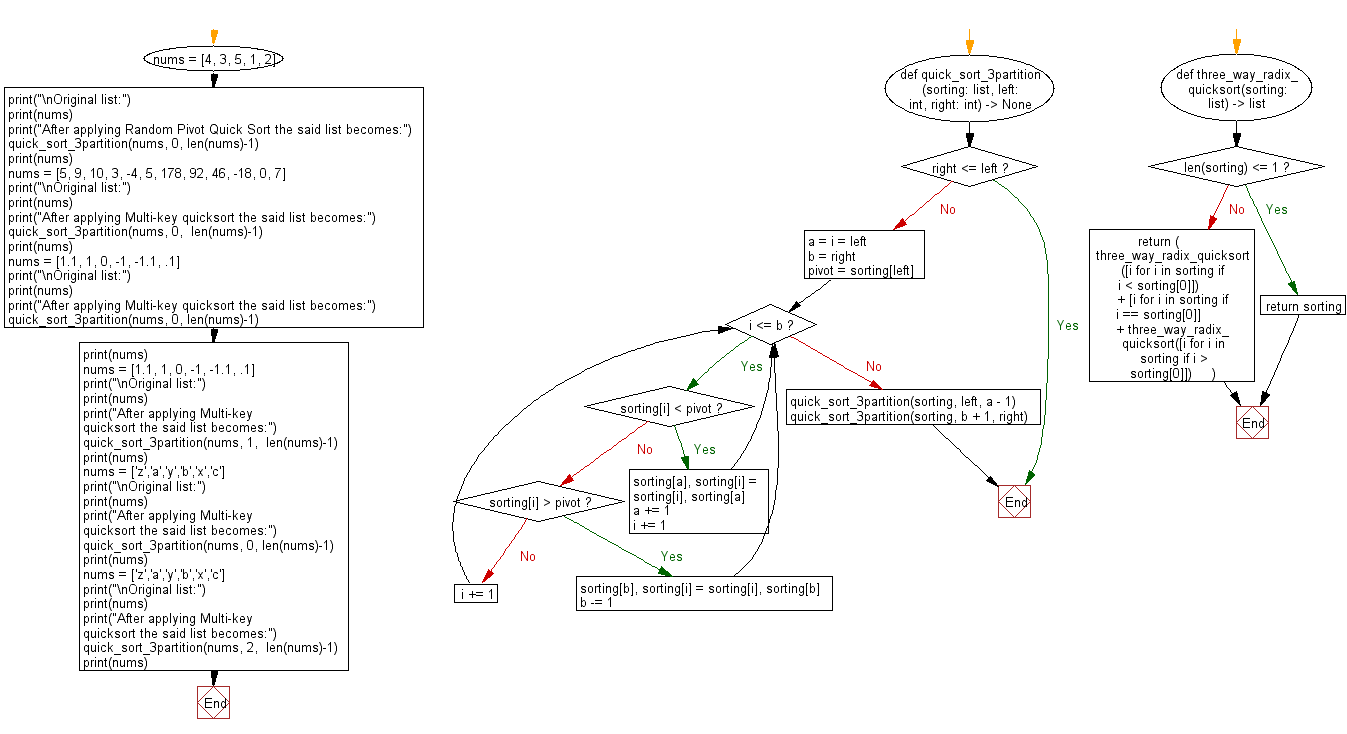﻿ Python: Sort unsorted numbers using Multi-key quicksort - w3resource# Python: Sort unsorted numbers using Multi-key quicksort

## Python Search and Sorting : Exercise-32 with Solution

Write a Python program to sort unsorted numbers using Multi-key quicksort.

From Wikipedia-
Multi-key quicksort:
This algorithm is a combination of radix sort and quicksort. Pick an element from the array (the pivot) and consider the first character (key) of the string (multikey). Partition the remaining elements into three sets: those whose corresponding character is less than, equal to, and greater than the pivot's character. Recursively sort the "less than" and "greater than" partitions on the same character.

Sample Solution:

Python Code:

``````#Ref.https://bit.ly/36fvcEw
def quick_sort_3partition(sorting: list, left: int, right: int) -> None:
if right <= left:
return
a = i = left
b = right
pivot = sorting[left]
while i <= b:
if sorting[i] < pivot:
sorting[a], sorting[i] = sorting[i], sorting[a]
a += 1
i += 1
elif sorting[i] > pivot:
sorting[b], sorting[i] = sorting[i], sorting[b]
b -= 1
else:
i += 1
quick_sort_3partition(sorting, left, a - 1)
quick_sort_3partition(sorting, b + 1, right)
if len(sorting) <= 1:
return sorting
return (
three_way_radix_quicksort([i for i in sorting if i < sorting])
+ [i for i in sorting if i == sorting]
+ three_way_radix_quicksort([i for i in sorting if i > sorting])
)
nums = [4, 3, 5, 1, 2]
print("\nOriginal list:")
print(nums)
print("After applying Random Pivot Quick Sort the said list becomes:")
quick_sort_3partition(nums, 0, len(nums)-1)
print(nums)
nums = [5, 9, 10, 3, -4, 5, 178, 92, 46, -18, 0, 7]
print("\nOriginal list:")
print(nums)
print("After applying Multi-key quicksort the said list becomes:")
quick_sort_3partition(nums, 0,  len(nums)-1)
print(nums)
nums = [1.1, 1, 0, -1, -1.1, .1]
print("\nOriginal list:")
print(nums)
print("After applying Multi-key quicksort the said list becomes:")
quick_sort_3partition(nums, 0, len(nums)-1)
print(nums)
nums = [1.1, 1, 0, -1, -1.1, .1]
print("\nOriginal list:")
print(nums)
print("After applying Multi-key quicksort the said list becomes:")
quick_sort_3partition(nums, 1,  len(nums)-1)
print(nums)
nums = ['z','a','y','b','x','c']
print("\nOriginal list:")
print(nums)
print("After applying Multi-key quicksort the said list becomes:")
quick_sort_3partition(nums, 0, len(nums)-1)
print(nums)
nums = ['z','a','y','b','x','c']
print("\nOriginal list:")
print(nums)
print("After applying Multi-key quicksort the said list becomes:")
quick_sort_3partition(nums, 2,  len(nums)-1)
print(nums)
```
```

Sample Output:

```Original list:
[4, 3, 5, 1, 2]
After applying Random Pivot Quick Sort the said list becomes:
[1, 2, 3, 4, 5]

Original list:
[5, 9, 10, 3, -4, 5, 178, 92, 46, -18, 0, 7]
After applying Multi-key quicksort the said list becomes:
[-18, -4, 0, 3, 5, 5, 7, 9, 10, 46, 92, 178]

Original list:
[1.1, 1, 0, -1, -1.1, 0.1]
After applying Multi-key quicksort the said list becomes:
[-1.1, -1, 0, 0.1, 1, 1.1]

Original list:
[1.1, 1, 0, -1, -1.1, 0.1]
After applying Multi-key quicksort the said list becomes:
[1.1, -1.1, -1, 0, 0.1, 1]

Original list:
['z', 'a', 'y', 'b', 'x', 'c']
After applying Multi-key quicksort the said list becomes:
['a', 'b', 'c', 'x', 'y', 'z']

Original list:
['z', 'a', 'y', 'b', 'x', 'c']
After applying Multi-key quicksort the said list becomes:
['z', 'a', 'b', 'c', 'x', 'y']
```

Flowchart:Python Code Editor:

What is the difficulty level of this exercise?

Test your Programming skills with w3resource's quiz.

﻿

## Python: Tips of the Day

The Zip() Function:

```>>> students = ('John', 'Mary', 'Mike')
>>> ages = (15, 17, 16)
>>> scores = (90, 88, 82, 17, 14)
>>> for student, age, score in zip(students, ages, scores):
...     print(f'{student}, age: {age}, score: {score}')
...
John, age: 15, score: 90
Mary, age: 17, score: 88
Mike, age: 16, score: 82
>>> zipped = zip(students, ages, scores)
>>> a, b, c = zip(*zipped)
>>> print(b)
(15, 17, 16)
```